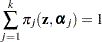Notation for the Finite Mixture Model

The general expression for the finite mixture model fitted with the FMM procedure is as follows: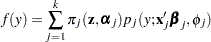The number of components in the mixture is denoted as. The mixture probabilities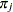can depend on regressor variablesand parameters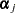. By default, the FMM procedure models these probabilities using a logit transform if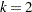and as a generalized logit model if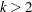. The component distributions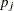can also depend on regressor variables in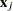, regression parameters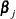, and possibly scale parameters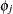. Notice that the component distributionsare indexed bysince the distributions might belong to different families. For example, in a two-component model, you might model one component as a normal (Gaussian) variable and the second component as a variable with adistribution with low degrees of freedom to manage overdispersion.

The mixture probabilitiessatisfy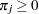, for all, and